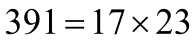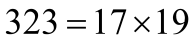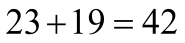A school has divided its 391 boys and 323 girls into the most equal classes possible, ensuring that the number of boys in each class is the same as that of the girls. How many classes are there in total?

# A school has divided its 391 boys and 323 girls into the most equal classes possible, ensuring that the number of boys in each class is the same as that of the girls. How many classes are there in total?

1. A
38
2. B
42
3. C
46
4. D
52

Fill Out the Form for Expert Academic Guidance!l

+91

Live ClassesBooksTest SeriesSelf Learning

Verify OTP Code (required)

### Solution:

Concept: The numbers will be expressed as the sum of their prime factors. Next, locate their HCF. The HCF of two numbers will represent how many pupils are in each class. Next, calculate the number of classes for males by dividing the number of boys by their HCF. Do the same for courses for girls. The total number of classes can then be determined by adding the two numbers of classes.
There are 391 boys and 323 girls in all.
By calculating the HCF of the numbers 391 and 323, we can determine how many students will be in each class.
We shall first represent the numbers as the product of the prime factors in order to calculate HCF.
 17 391 23 23 1
That is,17 323 19 19 1
Hence,Consequently, 17 is the biggest common factor between 391 and 323.
Thus, there will be 17 guys and 17 girls in each class.
When there are 391 boys, we will now determine how many classes of boys will be formed.
391 will be divided by 17Similarly, multiply the number of girls' classes by 17 to see how many classes will be produced when there are 323 girls.The total number of classes will then be determined by adding the two numbers of classes.Hence, the correct option is 2) 42

## Related content

 Area of Square Area of Isosceles Triangle Pythagoras Theorem Triangle Formula Perimeter of Triangle Formula Area Formulae Volume of Cone Formula Matrices and Determinants_mathematics Critical Points Solved Examples Type of relations_mathematics+91

Live ClassesBooksTest SeriesSelf Learning

Verify OTP Code (required)